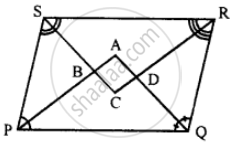# In the Following Diagram, the Bisectors of Interior Angles of the Parallelogram Pqrs Enclose a Quadrilateral Abcd. - Mathematics

Sum

In the following diagram, the bisectors of interior angles of the parallelogram PQRS enclose a quadrilateral ABCD.Show that:
(i) ∠PSB + ∠SPB = 90°
(ii) ∠PBS = 90°
(iii) ∠ABC = 90°
(v) ∠A = 90°
(vi) ABCD is a rectangle
Thus, the bisectors of the angles of a parallelogram enclose a rectangle.

#### Solution

Given: In parallelogram ABCD bisector of angles P and Q, meet at A, bisectors of ∠R and ∠S meet at C. Forming a quadrilateral ABCD as shown in the figure.

To prove :
(i) ∠PSB + ∠SPB = 90°
(ii) ∠PBS = 90°
(iii) ∠ABC = 90°
(v) ∠A = 9°
(vi) ABCD is a rectangle

Proof : In parallelogram PQRS,

PS || QR (opposite sides)

∠P +∠Q = 180°

and AP and AQ are the bisectors of consecutive angles

∠P and ∠Q of the parallelogram

∠APQ + ∠AQP = 1/2 x 180° = 90°

But in ∆APQ,

∠A + ∠APQ + ∠AQP = 180° (Angles of a triangle)

∠A + 90° = 180°

∠A = 180° – 90°

(v) ∠A = 90°

Similarly PQ || SR

∠PSB + SPB = 90°

(ii) and ∠PBS = 90°

But, ∠ABC = ∠PBS (Vertically opposite angles)

(iii) ∠ABC = 90°

Similarly we can prove that

(iv) ∠ADC = 90° and ∠C = 90°

(vi) ABCD is a rectangle (Each angle of a quadrilateral is 90°)

Hence proved.

Concept: Types of Quadrilaterals - Properties of a Parallelogram
Is there an error in this question or solution?

#### APPEARS IN

Selina Concise Mathematics Class 8 ICSE
Chapter 17 Special Types of Quadrilaterals
Exercise 17 | Q 16 | Page 199